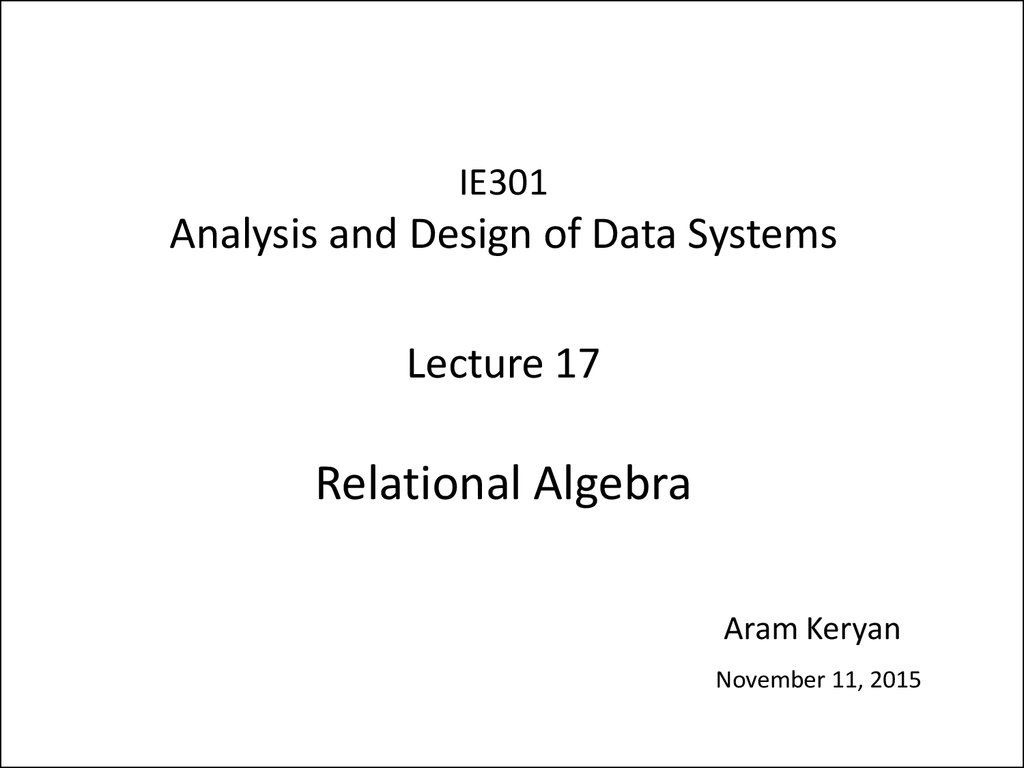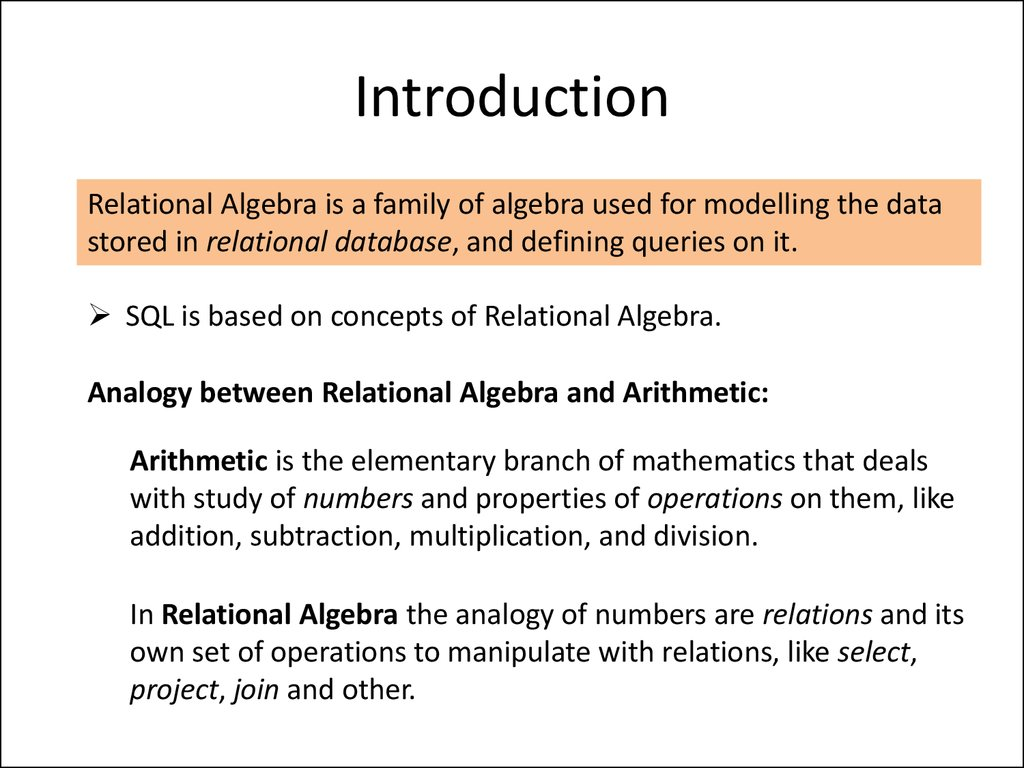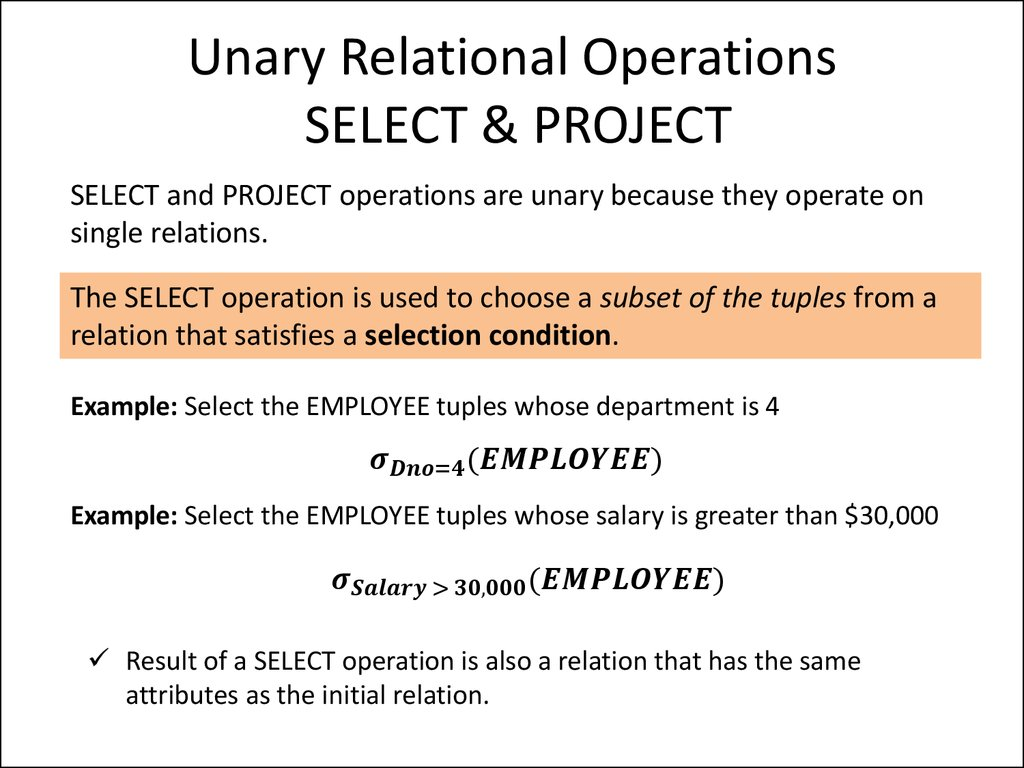# Analysis and Design of Data Systems. Relational Algebra (Lecture 17)

## 1.

IE301
Analysis and Design of Data Systems
Lecture 17
Relational Algebra
Aram Keryan
November 11, 2015

## 2. Introduction

Relational Algebra is a family of algebra used for modelling the data
stored in relational database, and defining queries on it.
SQL is based on concepts of Relational Algebra.
Analogy between Relational Algebra and Arithmetic:
Arithmetic is the elementary branch of mathematics that deals
with study of numbers and properties of operations on them, like